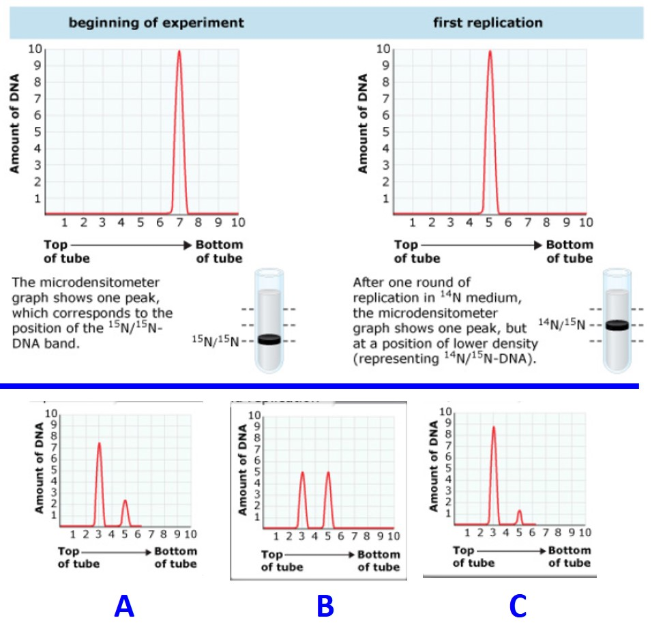# Problem: To confirm the semiconservative model of replication, it was important for Meselson and Stahl to quantify the amount of DNA in each band produced by density-gradient centrifugation. To accomplish this, they took advantage of the fact that DNA absorbs ultraviolet light, and used UV light to photograph each tube. By scanning the UV photographs with a microdensitometer, graphs like the ones below were produced. The height of each peak in the graph is directly proportional to the concentration of DNA in the corresponding band. Also, the position of each peak reflects the 14N and 15N content of the band. Suppose that the scientists analyzed the same amount of DNA (10 units) by density-gradient centrifugation after two, three, and four rounds of replication in 14N medium. What would you predict the microdensitometer graph would look like after each round? The beginning of the experiment as well as Replication Cycle 1 is given to you. Match up replication rounds “A”, “B” and “C” in the correct order with Replication Cycles 2, 3 and 4.Match up replication rounds “A”, “B” and “C” in the correct order with Replication Cycles 2, 3 and 4.a) replication cycle 2 =b) replication cycle 3 =c) replication cycle 4 =

###### Problem Details

To confirm the semiconservative model of replication, it was important for Meselson and Stahl to quantify the amount of DNA in each band produced by density-gradient centrifugation. To accomplish this, they took advantage of the fact that DNA absorbs ultraviolet light, and used UV light to photograph each tube. By scanning the UV photographs with a microdensitometer, graphs like the ones below were produced. The height of each peak in the graph is directly proportional to the concentration of DNA in the corresponding band. Also, the position of each peak reflects the 14N and 15N content of the band. Suppose that the scientists analyzed the same amount of DNA (10 units) by density-gradient centrifugation after two, three, and four rounds of replication in 14N medium. What would you predict the microdensitometer graph would look like after each round? The beginning of the experiment as well as Replication Cycle 1 is given to you. Match up replication rounds “A”, “B” and “C” in the correct order with Replication Cycles 2, 3 and 4.Match up replication rounds “A”, “B” and “C” in the correct order with Replication Cycles 2, 3 and 4.

a) replication cycle 2 =

b) replication cycle 3 =

c) replication cycle 4 =

Frequently Asked Questions

What scientific concept do you need to know in order to solve this problem?

Our tutors have indicated that to solve this problem you will need to apply the Meselson-Stahl Experiment concept. You can view video lessons to learn Meselson-Stahl Experiment. Or if you need more Meselson-Stahl Experiment practice, you can also practice Meselson-Stahl Experiment practice problems.

What is the difficulty of this problem?

Our tutors rated the difficulty ofTo confirm the semiconservative model of replication, it was...as high difficulty.

How long does this problem take to solve?

Our expert Biology tutor, Kaitlyn took 11 minutes and 30 seconds to solve this problem. You can follow their steps in the video explanation above.

What professor is this problem relevant for?

Based on our data, we think this problem is relevant for Professor Waletzko's class at OSU.